## Introduction to Searching Algorithms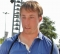Updated on 30-Jul-2019 22:30:23
The searching algorithms are used to search or find one or more than one element from a dataset. These type of algorithms are used to find elements from a specific data structures. Searching may be sequential or not. If the data in the dataset are random, then we need to use sequential searching. Otherwise we can use other different techniques to reduce the complexity. In this Section We are going to cover − Binary Search Exponential Search Interpolation Search Jump Search Linear Search Ternary Search

## Ternary Search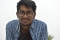Updated on 15-Jun-2020 14:50:10
Like the binary search, it also separates the lists into sub-lists. This procedure divides the list into three parts using two intermediate mid values. As the lists are divided into more subdivisions, so it reduces the time to search a key value.The complexity of Ternary Search TechniqueTime Complexity: O(log3 n)Space Complexity: O(1)Input and OutputInput: A sorted list of data: 12 25 48 52 67 79 88 93 The search key 52 Output: Item found at location: 3AlgorithmternarySearch(array, start, end, key)Input − An sorted array, start and end location, and the search keyOutput − location of the key (if found), otherwise wrong ... Read More

## Linear Search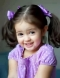Updated on 15-Jun-2020 14:54:10
Linear searching techniques are the simplest technique. In this technique, the items are searched one by one. This procedure is also applicable for unsorted data set. Linear search is also known as sequential search. It is named as linear because its time complexity is of the order of n O(n).The complexity of Linear Search TechniqueTime Complexity: O(n)Space Complexity: O(1)Input and OutputInput: A list of data: 20 4 89 75 10 23 45 69 the search key 10 Output: Item found at location: 4AlgorithmlinearSearch(array, size, key)Input − An sorted array, size of the array and the search keyOutput − location of the ... Read More

## Jump SearchUpdated on 15-Jun-2020 14:00:54
Jump search technique also works for ordered lists. It creates a block and tries to find the element in that block. If the item is not in the block, it shifts the entire block. The block size is based on the size of the list. If the size of the list is n then block size will be √n. After finding a correct block it finds the item using a linear search technique. The jump search lies between linear search and binary search according to its performance.The complexity of Jump Search TechniqueTime Complexity: O(√n)Space Complexity: O(1)Input and OutputInput: A sorted ... Read More

## Interpolation SearchUpdated on 15-Jun-2020 14:05:21
For the binary search technique, the lists are divided into equal parts. For the interpolation searching technique, the procedure will try to locate the exact position using interpolation formula. After finding the estimated location, it can separate the list using that location. As it tries to find exact location every time, so the searching time reduces. This technique can find items easily if the items are uniformly distributed.The complexity of Interpolation Search TechniqueTime Complexity: O(log2(log2 n)) for the average case, and O(n) for the worst case (when items are distributed exponentially)Space Complexity: O(1)Input and OutputInput: A sorted list of data: ... Read More

## Exponential Search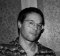Updated on 15-Jun-2020 14:10:42
Exponential search is also known as doubling or galloping search. This mechanism is used to find the range where the search key may present. If L and U are the upper and lower bound of the list, then L and U both are the power of 2. For the last section, the U is the last position of the list. For that reason, it is known as exponential.After finding the specific range, it uses the binary search technique to find the exact location of the search key.The complexity of Exponential Search TechniqueTime Complexity: O(1) for the best case. O(log2 i) ... Read More

## Binary Search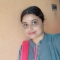Updated on 15-Jun-2020 14:14:41
When the list is sorted we can use the binary search technique to find items on the list. In this procedure, the entire list is divided into two sub-lists. If the item is found in the middle position, it returns the location, otherwise jumps to either left or right sub-list and do the same process again until finding the item or exceed the range.The complexity of Binary Search TechniqueTime Complexity: O(1) for the best case. O(log2 n) for average or worst case.Space Complexity: O(1) Input and OutputInput:  A sorted list of data: 12 25 48 52 67 79 88 93 The ... Read More